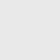# Operational Amplifier Gain Calculator

Easily calculate operational amplifier gain by using our calculator TO-Tools. In addition, we have two different calculators for non-inverting and inverting configurations OP-AMP.

## NON-Inverting Amplifier Gain CalculatorIn a non-inverting configuration, the input signal is given to a non-inverting terminal (+) of an OP-AMP. Thus, the output voltage will always be in-phase of the input signal applied.
The formula used for calculating the gain for a non-inverting opamp configuration is given below.

$G=1+\frac{R2}{R1}$

## Inverting Amplifier Gain CalculatorIn an inverting configuration, the input signal is given to an OP-AMP inverting terminal (-). As a result, the output voltage will always be inverted to the input signal applied, and therefore it is called a non-inverting amplifier.
The formula used for calculating the gain for an inverting opamp configuration is given below.

$G=-\frac{R2}{R1}$

## Usage

Here R2 is a feedback resistor, and R1 is an input resistor. You can check the above schematic for proper connection details for R2 and R1.
Now, insert any two values that you know, now press the calculate button, and our tool will calculate the third value. Thus, you can easily calculate the gain or any resistor value by using these tools.
For better understanding, the tool will also update the appropriate values in the schematic.
The tool will display the calculated result after round off at 3 decimal places if any.

If you are not familiar with an OP-AMP, we recommend having a look at our:
❑  Getting started guide on OP-AMP

## Subscribe to our weekly newsletterSubscribe
Notify ofInline Feedbacks

## Browse## Browse